Courses

# RD Sharma Solutions -Ex-10.2, Basic Geometrical Concepts, Class 6, Maths Class 6 Notes | EduRev

## RD Sharma Solutions for Class 6 Mathematics

Created by: Abhishek Kapoor

## Class 6 : RD Sharma Solutions -Ex-10.2, Basic Geometrical Concepts, Class 6, Maths Class 6 Notes | EduRev

The document RD Sharma Solutions -Ex-10.2, Basic Geometrical Concepts, Class 6, Maths Class 6 Notes | EduRev is a part of the Class 6 Course RD Sharma Solutions for Class 6 Mathematics.
All you need of Class 6 at this link: Class 6

Question 1. In the figure, points are given in two rows. Join the points AM ,HE, TO, RUN , IF . how many line segments are formed?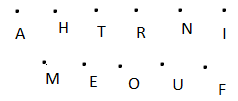If we join the points AM, HE , RUN , IF ,6 line segments can be formed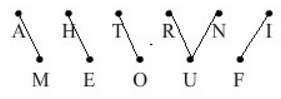These six line segments are AM, HE , RU , IF, UN

Question 2. In the figure name: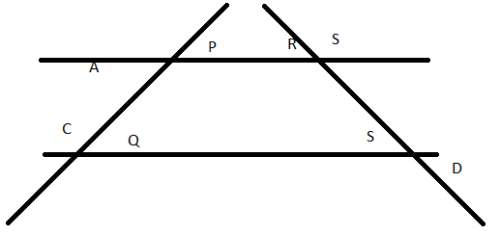i) Five line segments – PQ, RS, PR, QS, AP

ii) Five rays – QC->SD->PA->RB-> AND RA

iii) non intersecting line segments - PR , QS

Question 3. In each of the following cases, state whether you can draw line segments on the given surfaces:

i) The face of the cuboids

Yes, we can draw line segments on the face of the cuboids.

ii) The surface of an egg or apple

No, we cannot draw a line segment on the surface of an egg or apple

iii) The curved surface area of the cylinder

Four points such that there no three of them belong to the same line

Yes, we can draw line segments on the curved surface of a cylinder.

Every line segment parallel to the axis of a cylinder on the curved surface will be a line segment.

iv) The curved surface of the cone –

Yes we can draw line segments on the curved surface of the cone

Every line segment joining the vertex of a cone and any point on the circumference of the cone will be a line segment.

v) The base of the cone –

Yes , we can draw line segments on the base of the cone.

Yes, , we can draw line segments on the curved surface area of the cone.

Every line segment joining the vertex of a cone and any point on the circumference of the cone will form a line segment.

Question 4. Mark the following points on the sheet of the paper. Tell how many line segments can be obtained in the each case:

If there are n points in a plane and no three of them are collinear, the number of line segments obtained by joining these points is equal to n(n-1)2

On applying the above formula, we get ,

i) Two points A,B - number of line segments = 2(2-1) 2 = 1

ii) Three non – collinear points A,B,C

The number of line segments = 3(3-1) 2 = 3(22) = 3

iii) For four points such that no three of them belong to the same line

Number of line segments = 4(4-1)2 = 4(32) = 6

iv) Any five points so that no three of them are collinear

Number of line segments = 5(5-1) 2 = 5(42) = 10

Question 5. Count the number of line segments in figure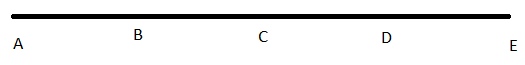Line segments in the given figure are AB, AC, AD, AE, BC, BD, BE, CD, CE and DE.

Thus, there are 10 line segments

Question 6. In the figure name all the rays with initial points as A, B, C respectively.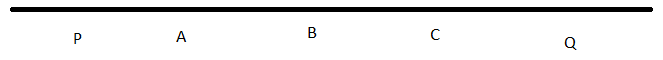Name of all the rays with initial point A :

AP, AB, AC, AQ

Name of all the rays with initial point B :

BP, BA, BC, BQ

Name of all the rays with initial point C :

CP, CA, CB , CQ

i) Is ray AB different from AC?

No, because the origin point of both the rays. AB, AC

ii) Is ray BA different from CA?

Yes, because the original point of both the rays. BA and CA are different

iii) Is ray CP different from ray CQ?

Yes, because both the rays. CP and CQ are different.

Question 7. Give three examples of line segments from the environment

Solution : Examples of line segments in our home are:

i) Grout lines in the tile floors

ii) Groves where wooden flooring connects

iii) Metal outline of a sliding glass door.

103 docs

,

,

,

,

,

,

,

,

,

,

,

,

,

,

,

,

,

,

,

,

,

,

,

,

,

,

,

,

,

,

;